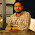Price elasticity of demand example - FreeEconHelp.com, Learning Economics... Solved!

## 7/30/18

Price elasticity of demand example question where you have to solve for the percent change in quantity or price instead of the elasticity measure. Imagine an elasticity question that gives you the elasticity and then asks you to calculate the percent change in either quantity or price given the percent change in the other term. For example, you are told that the price elasticity of demand for apples is -2 and that  quantity demanded of applies increases from 100 apples to 250 apples. What would the corresponding change in price have to be to accommodate this change in quantity demanded?

First we have to set up our standard price elasticity of demand formula or equation:

Price elasticity of Demand = The percent change in quantity demanded/the percent change in pricePrice elasticity of demand Equation
Using this equation from our examples above we will plug the -2 value into the equation as well as the percent change in quantity demanded is found by taking the difference between 100 and 250 apples and then dividing by the average (,1000+2,500)/2 --an example of the midpoints theorem in action-- which gives us 1,750. The result is 1,500 divided by 1,750 which is 6/7. Once we plug these values into the price elasticity of demand formula above we will get:

-2  = 6/7 / Percent change in price.

If we multiply both sides of the equation by percent change in price and divide both sides by -2 we end up with:

Percent change in price = -6/14

We can see that the change in price necessary to change the quantity demanded by 1,500 units would be a -6/14 change in price or a roughly 43% decrease in the price level for apples.Exploring the price elasticity of demand for apples
The trick to solving this type of elasticity problem is to remember the price elasticity of demand formula and plugging in all of the information that you have available and then performing some algebra to get the value that you are missing. For example, if you need to solve for the percent change in price, you should set up your elasticity formula  to look like:

Elasticity = X/Percent change in price

You can then multiply both sides by the percent change in price and then solve for X to get your answer.

Similarly, if the problem asks you to find the percent change in price, you can set up the elasticity equation to look like:

Elasticity = Percent change in quantity demanded/X

You then must multiply both sides by X and divide both sides by the elasticity measure in order to solve for X.

Let's consider another example where the elasticity measure is -3 and the percent change in price is equal to -16% (meaning that price falls by 16%). Using the methods discussed earlier in this post we know that we have to plug this information into our elasticity formula to find out what the percent change in quantity will be. This gives us the following elasticity formula:

-3 = X / -16%

First, we know that a price elasticity of demand measure of -3 is highly elastic so we would expect the corresponding percent change in quantity demanded to be much larger than the percent change in price of 16%. In fact, when we multiply both sides by -16% we end up with X equals to 48% which means that our quantity demanded will increase by almost 50% given a much lower percent change in price. This confirms that the price elasticity of demand measure for the good is very elastic and responsive to associated changes in price level.

1.2.3.